# Electronics and Communication Engineering - Analog Electronics

11.

In figure The minimum and maximum load currents are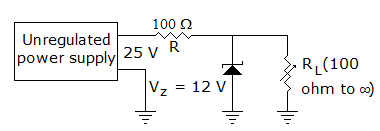A. 0 and 60 mA B. 0 and 120 mA C. 10 mA and 60 mA D. 10 mA and 120 mA

Explanation:

When RL = ∞, IL = 0,

When RL = 100 Ω,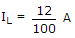or 120 mA.

12.

In figure, VEB = 0.6 V, β = 99. Then VC and IC are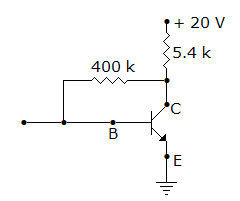A. 9.3 V and 1.98 mA respectively B. 4.6 V and 1.98 mA respectively C. 9.3 V and 0.02 mA respectively D. 4.6 V and 0.02 mA respectively

Explanation: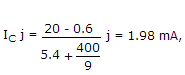VC = 20 - 1.98 x 10-3 x 5.4 x 103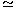9.3 V.

13.

The input impedance of op-amp circuit of figure is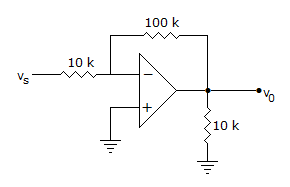A. 120 k ohm B. 110 k ohm C. infinity D. 10 k ohm

Explanation:

Due to the presence of virtual ground at input, the resistance in the series path of input of inverting amplifier is input impedance.

14.

In a BJT circuit a pnp transistor is replaced by npn transistor. To analyse the new circuit

 A. all calculations done earlier have to be repeated B. replace all calculated voltages by reverse values C. replace all calculated currents by reverse values D. replace all calculated voltages and currents by reverse values

Explanation:

All voltages and currents have reverse polarity.

15.

To protect the diodes in a rectifier and capacitor input filter circuit it is necessary to use

 A. surge resistor B. surge inductor C. surge capacitor D. both (a) and (b)